# Curve

In mathematics, the concept of a curve tries to capture the intuitive idea of a geometrical one-dimensional and continuous object. A simple example is the circle. In everyday use of the term "curve," a straight line is not curved, but in mathematical parlance curves include straight lines and line segments. A large number of other curves have been studied in geometry.

The term curve is also used in ways making it almost synonymous with mathematical function (as in learning curve), or graph of a function (Phillips curve).

## Conventions and terminology

It is important to distinguish between a curve and its image. Two distinct curves may have the same image. For example, a line segment can be traced out at different speeds, or a circle can be traversed a different number of times. Often, however, we are just interested in the image of the curve. It is important to pay attention to context and convention when reading about curves.

Terminology is also not uniform. Topologists often use the term "path" for what we call a curve, and "curve" for what we call the image of a curve. The term "curve" is more common in vector calculus and differential geometry.

## History

A curve may be a locus, or a path. That is, it may be a graphical representation of some property of points; or it may be traced out, for example by a stick in the sand on a beach. Of course, if one says curved in ordinary language, it means bent (not straight), so refers to a locus. This leads to the general idea of curvature. Since the formulation of Newtonian dynamics, we have come to understand that for an object to follow a curved path, it must experience acceleration. This understanding is important because major examples of curves are the orbits of planets. One reason for the use of the Ptolemaic system of epicycles and deferents was the special status accorded to the circle as curve.

The conic sections had been studied in depth by Apollonius of Perga. They were applied in astronomy by Kepler. The Greek geometers had studied many other kinds of curves. One reason was their interest in geometric constructions, going beyond compass and straightedge. In that way, the intersection of curves could be used to solve some polynomial equations, such as that involved in trisecting an angle.

Newton also worked on an early example in the calculus of variations. Solutions to variational problems, such as the brachistochrone and tautochrone questions, introduced properties of curves in new ways (in this case, the cycloid). The catenary gets its name as the solution to the problem of a hanging chain, the sort of question that became routinely accessible by means of differential calculus.

In the eighteenth century came the beginnings of the theory of plane algebraic curves, in general. Newton had studied the cubic curves, in the general description of the real points into "ovals." The statement of Bézout's theorem showed a number of aspects that were not directly accessible to the geometry of the time, to do with singular points and complex solutions.

From the nineteenth century, there is not a separate curve theory, but rather the appearance of curves as the one-dimensional aspect of projective geometry, and differential geometry; and later topology, when for example the Jordan curve theorem was understood to lie quite deep, as well as being required in complex analysis. The era of the space-filling curves finally provoked the modern definitions of curve.

## Mathematical definitions

In mathematics, a (topological) curve is defined as follows. Let$I$ be an interval of real numbers (i.e. a non-empty connected subset of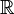$\mathbb{R}$). Then a curve$\!\,\gamma$ is a continuous mapping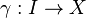$\,\!\gamma : I \rightarrow X$, where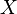$X$ is a topological space. The curve$\!\,\gamma$ is said to be simple if it is injective, i.e. if for all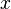$x$,$y$ in$I$, we have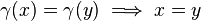$\,\!\gamma(x) = \gamma(y) \implies x = y$. If$I$ is a closed bounded interval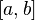$\,\![a, b]$, we also allow the possibility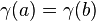$\,\!\gamma(a) = \gamma(b)$ (this convention makes it possible to talk about closed simple curve). If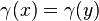$\gamma(x)=\gamma(y)$ for some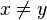$x\ne y$ (other than the extremities of$I$), then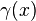$\gamma(x)$ is called a double (or multiple) point of the curve.

A curve$\!\,\gamma$ is said to be closed or a loop if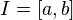$\,\!I = [a, b]$ and if$\!\,\gamma(a) = \gamma(b)$. A closed curve is thus a continuous mapping of the circle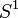$S^1$; a simple closed curve is also called a Jordan curve.

A plane curve is a curve for which X is the Euclidean plane — these are the examples first encountered—or in some cases the projective plane. A space curve is a curve for which X is of three dimensions, usually Euclidean space; a skew curve is a space curve which lies in no plane. These definitions also apply to algebraic curves (see below). However, in the case of algebraic curves it is very common not to restrict the curve to having points only defined over the real numbers.

This definition of curve captures our intuitive notion of a curve as a connected, continuous geometric figure that is "like" a line, without thickness and drawn without interruption, although it also includes figures that can hardly be called curves in common usage. For example, the image of a curve can cover a square in the plane (space-filling curve). The image of simple plane curve can have Hausdorff dimension bigger than one (see Koch snowflake) and even positive Lebesgue measure (the last example can be obtained by small variation of the Peano curve construction). The dragon curve is yet another weird example.

## Lengths of curves

Main article: arc length

If$X$ is a metric space with metric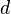$d$, then we can define the length of a curve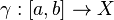$\!\,\gamma : [a, b] \rightarrow X$ by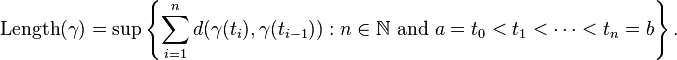$\mbox{Length} (\gamma)=\sup \left\{ \sum_{i=1}^n d(\gamma(t_i),\gamma(t_{i-1})) : n \in \mathbb{N} \mbox{ and } a = t_0 < t_1 < \cdots < t_n = b \right\}.$

A rectifiable curve is a curve with finite length. A parametrization of$\!\,\gamma$ is called natural (or unit speed or parametrised by arc length) if for any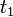$t_1$,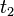$t_2$ in$[a, b]$, we have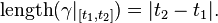$\mbox{length} (\gamma|_{[t_1,t_2]})=|t_2-t_1|.$

If$\!\,\gamma$ is a Lipschitz-continuous function, then it is automatically rectifiable. Moreover, in this case, one can define speed of$\!\,\gamma$ at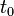$t_0$ as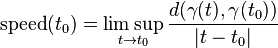$\mbox{speed}(t_0)=\limsup_{t\to t_0} {d(\gamma(t),\gamma(t_0))\over |t-t_0|}$

and then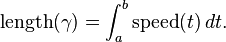$\mbox{length}(\gamma)=\int_a^b \mbox{speed}(t) \, dt.$

In particular, if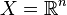$X = \mathbb{R}^n$ is Euclidean space and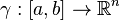$\gamma : [a, b] \rightarrow \mathbb{R}^n$ is differentiable then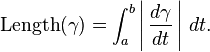$\mbox{Length}(\gamma)=\int_a^b \left| \, {d\gamma \over dt} \, \right| \, dt.$

## Differential geometry

While the first examples of curves that are met are mostly plane curves (that is, in everyday words, curved lines in two-dimensional space), there are obvious examples such as the helix which exist naturally in three dimensions. The needs of geometry, and also for example classical mechanics are to have a notion of curve in space of any number of dimensions. In general relativity, a world line is a curve in spacetime.

If$X$ is a differentiable manifold, then we can define the notion of differentiable curve in$X$. This general idea is enough to cover many of the applications of curves in mathematics. From a local point of view one can take$X$ to be Euclidean space. On the other hand it is useful to be more general, in that (for example) it is possible to define the tangent vectors to$X$ by means of this notion of curve.

If$X$ is a smooth manifold, a smooth curve in$X$ is a smooth map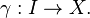$\!\,\gamma : I \rightarrow X.$

This is a basic notion. There are less and more restricted ideas, too. If$X$ is a$C^k$ manifold (i.e., a manifold whose charts are$k$ times continuously differentiable), then a$C^k$ curve in$X$ is such a curve which is only assumed to be$C^k$ (i.e.$k$ times continuously differentiable). If$X$ is an analytic manifold (i.e. infinitely differentiable and charts are expressible as power series), and$\!\,\gamma$ is an analytic map, then$\!\,\gamma$ is said to be an analytic curve.

A differentiable curve is said to be regular if its derivative never vanishes. (In words, a regular curve never slows to a stop or backtracks on itself.) Two$C^k$ differentiable curves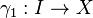$\!\,\gamma_1 :I \rightarrow X$ and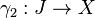$\!\,\gamma_2 : J \rightarrow X$

are said to be equivalent if there is a bijective$C^k$ map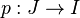$\!\,p : J \rightarrow I$

such that the inverse map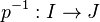$\!\,p^{-1} : I \rightarrow J$

is also$C^k$, and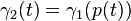$\!\,\gamma_{2}(t) = \gamma_{1}(p(t))$

for all$t$. The map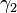$\!\,\gamma_2$ is called a reparametrisation of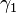$\!\,\gamma_1$; and this makes an equivalence relation on the set of all$C^k$ differentiable curves in$X$. A$C^k$ arc is an equivalence class of$C^k$ curves under the relation of reparametrisation.

## Algebraic curve

Algebraic curves are the curves considered in algebraic geometry. A plane algebraic curve is the locus of points f(x, y) = 0, where f(x, y) is a polynomial in two variables defined over some field F. Algebraic geometry normally looks at such curves in the context of algebraically closed fields. If K is the algebraic closure of F, and C is a curve defined by a polynomial f(x, y) defined over F, the points of the curve defined over F, consisting of pairs (a, b) with a and b in F, can be denoted C(F); the full curve itself being C(K).

Algebraic curves can also be space curves, or curves in even higher dimensions, obtained as the intersection (common solution set) of more than one polynomial equation in more than two variables. By eliminating variables by means of the resultant, these can be reduced to plane algebraic curves, which, however, may introduce singularities such as cusps or double points. We may also consider these curves to have points defined in the projective plane; if f(x, y) = 0 then if x = u/w and y = v/w, and n is the total degree of f, then by expanding out wnf(u/w, v/w) = 0 we obtain g(u, v, w) = 0, where g is homogeneous of degree n. An example is the Fermat curve un + vn = wn, which has an affine form xn + yn = 1.

Important examples of algebraic curves are the conics, which are nonsingular curves of degree two and genus zero, and elliptic curves, which are nonsingular curves of genus one studied in number theory and which have important applications to cryptography. Because algebraic curves in fields of characteristic zero are most often studied over the complex numbers, algbebraic curves in algebraic geometry look like real surfaces. Looking at them projectively, if we have a nonsingular curve in n dimensions, we obtain a picture in the complex projective space of dimension n, which corresponds to a real manifold of dimension 2n, in which the curve is an embedded smooth and compact surface with a certain number of holes in it, the genus. In fact, non-singular complex projective algebraic curves are compact Riemann surfaces.